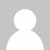The difficulty level has been slowly ramping up in this year’s Advent of Code challenge, and today’s puzzle initially sent me off down a dead end.

Part 1 was simple enough. We’re given a series of “dance moves”, involving rearranging the order of a line of dancers. First of all I needed to parse the instructions, which I did with a regex:

``````let instructions = input.split(',')
.map(i => i.match(/x(\d+)\/(\d+)|s(\d+)|p([a-z])\/([a-z])/))
.map(([x,a,b,c,d,e]) => ({move:x,exA:Number(a),exB:Number(b),spin:Number(c),pA:d,pB:e}))
``````

We needed two helpers to implement the three dance moves. “Exchange” swaps the position of two dancers, while “spin” moves a group of dancers from the end of the line to the front:

``````let exchange = (state,a,b) => {
let tmp = state[a]
state[a] = state[b]
state[b] = tmp
return state;
}
let spin = (state,n) => {
return state.slice(state.length - n).concat(state.slice(0,state.length - n))
}
``````

We can now simply iterate through the instructions and apply them, turning the final state back into a string:

``````function dance(instructions, start) {
let s = [...start]
for(let i of instructions) {
switch(i.move) {
case "x": s = exchange(s,i.exA,i.exB); break;
case "s": s = spin(s,i.spin); break;
case "p": s = exchange(s,s.indexOf(i.pA), s.indexOf(i.pB)); break;
}
}
return s.join('')
}
``````

So far so good. But for part 2 we were asked to repeat the dance 1 billion times. A quick calculation from the time taken to solve part 1 revealed this would take 2117 hours! So I knew I couldn’t just optimise my part 1 solution – even a hundredfold speedup would be way too slow.

I realised there must be a way to short-circuit the problem. My first idea was that maybe after a certain number of dances, we would get back to the starting point. So if after 7 dances we are back to the starting position then after 7 million dances we will be in the same position again. However, when I considered how many possible orderings of dancers there were (16 factorial by my calculation), I abandoned this idea.

My second idea was that maybe the instructions for one dance could be collapsed into a simple reordering. Maybe at the end of the dance the dancer who started in position 1 always ends up in position 6, and so on for each dancer.

So I took the answer for part 1 and generated a lookup of starting to end position for each dancer. And then I reapplied it one billion times:

``````let m = new Map()
let part1 = "pkgnhomelfdibjac"
for(let n = 0; n < state.length; n++) {
m.set(n, part1.indexOf(state[n]))
}
state = [..."abcdefghijklmnop"]
for(let n = 0; n < 1000000000; n++) {
let next = []
for (let i = 0; i < 16; i++) {
next[m.get(i)] = state[i]
}
state = next;
}
return state.join('')
``````

This took three minutes to run, but it generated the wrong answer. And on reflection, it wasn’t hard to see why this was never going to work. The “partner” dance move swapped dancers based on their original position, not their current position, so this optimization is way too simplistic.

So it was back to the drawing board, and it turns out that my first idea was the better one. If we repeatedly perform the dance, and wait until we see the starting state again, we’ve found the cycle length. From the cycle length we know how many additional dances are needed to make it up to one billion:

``````let cur = start;
let repetitions = 1000000000;
for(let n = 0; n < repetitions; n++) {
cur = dance(instructions,cur);
if (cur === start) {
let cycle = n+1;
let extras = repetitions % cycle;
n = 0;
repetitions = extras+1;
}
}
return cur
``````

With this optimization in place, I get the solution in a very respectable 300ms. The cycle size for my input was just 60 meaning only 40 extra repetitions were required. However, we could speed that up even further by storing the output for each dance, so that once we’d found the cycle, we could just return the answer we calculated from the first time through the cycle. That shaves it down to 200ms and looks like this (although I have just left my solution for part 2 as is):

``````let cur = start;
let solutions = []
let repetitions = 1000000000;
for(let n = 0; n < repetitions; n++) {
cur = dance(instructions,cur);
solutions.push(cur)
if (cur === start) {
let cycle = n+1;
let extras = repetitions % cycle;
return solutions[extras-1]
}
}
``````

So today’s challenge was a good lesson in optimization techniques, and a reminder not to be too quick to dismiss your initial ideas. I was wrong about the total number of possible orderings of dancers – its not 16 factorial (20,922,789,888,000), its a more reasonable 140 squared (19,600) – courtesy of the mathematical geniuses on the AoC reddit. And in any case just because the number of possible orderings is high, doesn’t mean the cycle size was high. If I’d started off by searching for a cycle, with an upper bound of say 10,000 repetitions I would have wound up at the answer much quicker.